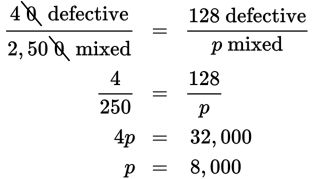# SAT Math Multiple Choice Question 518: Answer and Explanation

### Test Information

Question: 518

8. On average, for every 2,500 cans of colored paint a home improvement chain mixes, exactly 40 are the wrong color (defective). At this rate, how many cans of paint were mixed during a period in which exactly 128 were defective?

• A. 5,200
• B. 7,500
• C. 8,000
• D. 64,000

Explanation:

C

Difficulty: Easy

Category: Problem Solving and Data Analysis / Rates, Ratios, Proportions, and Percentages

Strategic Advice: When ratios involve large numbers, simplify if possible to make the calculations easier.

Getting to the Answer: Let p equal the number of cans of paint mixed. Set up a proportion and solve for p. Try writing the proportion in words first.